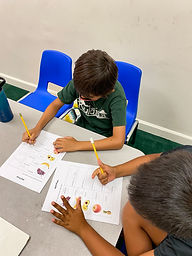## Ms. Kylie

### Target 1​

###### Lesson Type:

Continuation

Data Probability

:

Ratio

Use ratio understandings to identify a unit rate.

###### 1:

Solve unit rate problems for unit price.

###### 2:

Define rate, unit rate, and unit price.

###### 3:

Calculate unit rates.

6th

###### Vocabulary:

Unit Rate, Unit Price, Ratio, Rate

Activities:

-Students practiced finding the unit rate of ratio word problems

-Students learned to find the unit price of a single item. They were given a picture visual of an item and practiced finding the unit price using the rate that was given### Home Exploration

###### Guiding Questions:## Absent Students:

### Target 2

:

###### 1:

Use the corresponding sides of similar triangles to determine the unknown length of a side.

###### 2:

Find the ratio of corresponding sides and use that ratio to find unknown lengths.

6th

###### Vocabulary:

Proportional Relationship, Corresponding Sides

Activities:

-Students were given several different pairs of similar triangles that had a missing length. They found the ratio of corresponding sides to help find the missing length.### Home Exploration

###### Guiding Questions:### Target 3

:

###### Vocabulary:

Activities:### Home Exploration